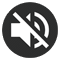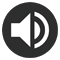# 11 Times table Chart - Printable PDF

6 Reviews

## Print the 11 Times table Chart

A pretty 11 Times table chart in A4 format (PDF) that will help you learn your 11 times table. Thanks to its colored numbers, it will make it easier for you to memorize the multiplication results.

Print for free this 11 times table and don't forget to take a look at the time-saving trick for the 11 times table. Then, practice online this times table. It will help you memorize the table even faster.

This chart is also available in other colors or in black and white. Feel free to look at all the templates we propose below and choose your favorite one!
In a Multiplication Chart, the results are displayed in a table (with columns and rows), whereas in a Times table Chart they are displayed as a list of increasing multiplications.

Here the list starts at 11x1 and ends at 11x12. One way to memorize your table is to recite it aloud in this way:

11 times 1... 11,  11 times 2... 22,  11 times 3... 33,  11 times 4... 44,  11 times 5... 55,  11 times 6... 66,  11 times 7... 77,  11 times 8... 88,  11 times 9... 99,  11 times 10... 110,  11 times 11... 121,  11 times 12... 132

This way of learning using auditory memory is effective for many children.

Print the 11 Times table Chart (PDF)

If you plan to print the other times tables, we advise you to choose a different color for each multiplication table from 1 to 12. To save time, you will find, in our All Times tables section, PDFs including all the tables colored like a rainbow.

## Our other 11 Times table PDF

Thanks to the many colors available, you will undoubtedly find your favorite chart! Our black and white 11 Times table charts will be perfect if you prefer sobriety, if you want to save ink or if you prefer to color your times table yourself using your pencils or markers. Feel free to click on the images to preview the PDF or use the "PRINT" button below the image to download the chart of your choice.

11 Times table Chart
Black & white
Print
11 Times table Chart
Handwritten numbers
Print
11 Times table Chart
Ink saving
Print
11 Times table Chart
Grey
Print
11 Times table Chart
Dark green
Print
11 Times table Chart
Green
Print
11 Times table Chart
Light green
Print
11 Times table Chart
Turquoise
Print
11 Times table Chart
Blue
Print
11 Times table Chart
Dark blue
Print
11 Times table Chart
Purple
Print
11 Times table Chart
Pink
Print
11 Times table Chart
Light pink
Print

## Trick for the 11 times table

You'll see that with our trick the 11 times table is a piece of cake! Not only will you easily memorize the multiplication 11x11 and 11x12, but you'll also be able to quickly find the result of a much more complex multiplication like, for example, 11 x 54. Check out our tips below and impress your friends ;)

### For the 11x1 to 11x9 multiplications

This case is very easy because the number by which we multiply 11 is doubled:

• 11 x 1 = 11
• 11 x 2 = 22
• 11 x 3 = 33
• 11 x 4 = 44
• 11 x 5 = 55
• 11 x 6 = 66
• 11 x 7 = 77
• 11 x 8 = 88
• 11 x 9 = 99

### For the 11x10 multiplication

The rule of the table of 10 applies: A number multiplied by 10 is always equal to the number followed by a zero:

11 x 10 = 110

### Pour les multiplications de 11x11 à 11x99

There's a simple rule that allows you to find the result without calculating it. When multiplying a 2-digit number by 11: space the 2 digits of the number and insert the sum of these 2 digits in the middle. There are 2 cases:

#### 1- If the sum of the 2 digits is less than 10:

• 11 x 11 = 121  (because 1 + 1 = 2)
• 11 x 12 = 132  (because 1 + 2 = 3)
• 11 x 13 = 143  (because 1 + 3 = 4)
• 11 x 14 = 154  (because 1 + 4 = 5)
• 11 x 15 = 165  (because 1 + 5 = 6)
• 11 x 16 = 176  (because 1 + 6 = 7)
• 11 x 17 = 187  (because 1 + 7 = 8)
• 11 x 18 = 198  (because 1 + 8 = 9)
• 11 x 20 = 220  (because 2 + 0 = 2)
• 11 x 21 = 231  (because 2 + 1 = 3)
• 11 x 22 = 242  (because 2 + 2 = 4)
• ...

#### 1- If the sum of the 2 digits is higher than 10:

It's the same as in the previous case, except that you add 1 to the 1st digit!

• 11 x 19 = 209  (because 1 + 9 = 10 and 1 + 1 = 2)
• 11 x 47 = 517  (because 4 + 7 = 11 and 4 + 1 = 5)
• 11 x 66 = 726  (because 6 + 6 = 12 and 6 + 1 = 7)
• ...
• 11 x 99 = 1089  (because 9 + 9 = 18 and 9 + 1 = 10)

## Practice the 11 times table

In this exercise, you can choose between several settings, so it's up to you to select the ones that suit you best. Here are the available options:

• Number color: By choosing the colorful numbers option, the color code of the table of 11 (orange) is applied. This is the same color code as the printable 11 times table chart at the top of the page. The use of color helps memorization, so we highly recommend the colorful mode!
• Number format: you can choose a specific font for the numbers. There are standard fonts, but also school fonts. Select the one that's closest to the one used at school.
• Game mode: random or increasing.

If you like this exercise, you can also practice the other tables in the All times tables section.

USEFUL !
You can see your more common mistakes below the settings.NUMBERS :
NUMBER FORMAT :
MULTIPLICATIONS :
GAME MODE :

### Your most common mistakes :

You will find here the list of your most common mistakes

### A chart with all the times tables (2 PDF to cut and assemble)

A pretty chart with all the times tables to print in A4 format (PDF). We have assigned a different color code for each times table, with a nice color gradient. The colors will help you memorize all your multiplication tables from the 1 to the 12 times table (other colors are available for this resource).

See the Chart